Latest Banking jobs   »   Quantitative Aptitude Quiz For Bank Mains...

# Quantitative Aptitude Quiz For Bank Mains Exams 2021- 25th January

Directions (1-5). Following are the questions based on two statements and answer the following based on the given statements.

Q1. What will be respective ratio of saving of Veer & Deepak.
Statement I . Income of Veer is 4% less than that of Sameer and also expenditure of Veer is 12.5% less than that of Sameer. Deepak spend 3/5 th of his income.
Statement II . Sameer save Rs. 7000 & Veer save Rs. 7400. Income of Deepak is Rs. 1000 more than that of Sameer.
(a) Only statement I is sufficient
(b) Only statement II is sufficient
(c) Statement I and II both together is sufficient
(d) Either statement I or Statement II alone is sufficient
(e) Neither statement I nor statement II is sufficient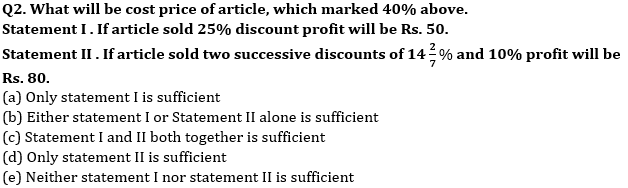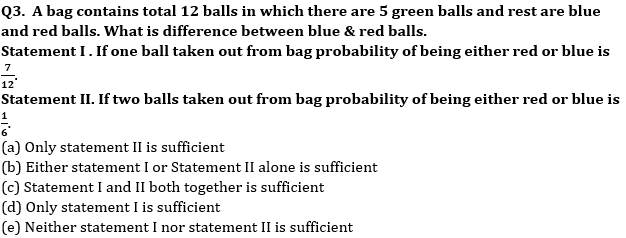Q4. Side of square is 3.5 cm more than radius of circle. What will be area of square?
Statement I. Difference between circumference and diameter of circle is 45 cm.
Statement II. Radius of circle is 50% more than breadth of rectangle whose length is 15 cm. Ratio of circumference of circle & perimeter of rectangle is 3 : 2.
(a) Only statement II is sufficient
(b) Either statement I or Statement II alone is sufficient
(c) Statement I and II both together is sufficient
(d) Only statement I is sufficient
(e) Neither statement I nor statement II is sufficient

Q5. What will be length of train A?
Statement I . Relative speed of train A & B is 10 meters/sec when both running in same direction and length of train B is 240 (Speed of train B is more than speed of train A).
Statement II . Train B cross a pole in 8 sec and cross train A in 12 sec running in opposite direction.
(a) Only statement II is sufficient
(b) Either statement I or Statement II alone is sufficient
(c) Neither statement I nor statement II is sufficient
(d) Only statement I is sufficient
(e) Statement I and II both together is sufficient

Directions (6- 7): The following questions are accompanied by two statements (I) and (II). You have to determine which statements(s) is/are sufficient/necessary to answer the questions.
(a) Statement (I) alone is sufficient to answer the question but statement (II) alone is not sufficient to answer the question.
(b) Statement (II) alone is sufficient to answer the question but statement (I) alone is not sufficient to answer the question.
(c) Both the statements taken together are necessary to answer the question, but neither of the statements alone is sufficient to answer the question.
(d) Either statement (I) or statement (II) by itself is sufficient to answer the question.
(e) Statements (I) and (II) taken together are not sufficient to answer the question.

Q6. A & B are two workers. If both A & B start work alternatively starting with A, then in how many hours work will be completed?
I. Efficiency of B is 50% more than A. If both A & B work together they can complete the task in 12 hours.
II. Ratio of efficiency of A : B is 2 : 3 and C is 25% more efficient than A. If C work for first hour and second hour all three work together and this process continued till the end, then work will be completed in 12 hours.

Q7. Total number of boys in school is how much more than total number of girls in school in 2016?
I. Total number of students in 2015 is 1152 and number of boys & girls increased by 20% and 25% in the year 2016 compared to year 2015.
II. If 50% of total number of boys and 75% of total number of girls appeared in the 2015 exam, then total number of girls appeared in the exam is 64 more than that of total number of boys appeared in the 2015 exam.

Directions (8 -10): The following questions are accompanied by two statements (I) and (II). You have to determine which statements(s) is/are sufficient/necessary to answer the questions.
(a) Statement (I) alone is sufficient to answer the question but statement (II) alone is not sufficient to answer the questions.
(b) Statement (II) alone is sufficient to answer the question but statement (I) alone is not sufficient to answer the question.
(c) Both the statements taken together are necessary to answer the questions, but neither of the statements alone is sufficient to answer the question.
(d) Either statement (I) or statement (II) by itself is sufficient to answer the question.
(e) Statements (I) and (II) taken together are not sufficient to answer the question.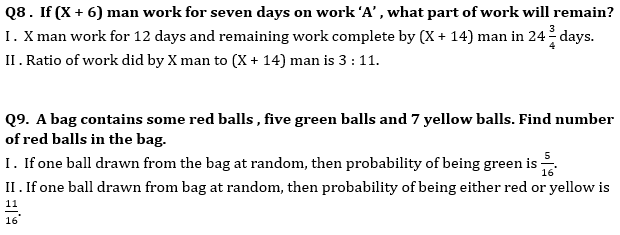Q10. A shopkeeper bought a jeans at the price of ‘2x’. Find the profit of shopkeeper (In Rs.)
I . Shopkeeper sold jeans at 20% profit after allowing two successive discounts of 20% & 4% respectively and difference between marked price and cost price is Rs. 810.
II . Cost price of a shirt is 75% of cost price of jeans and the difference of CP of shirt and CP of jeans is Rs. 360. Shopkeeper marks up jeans by 56.25% and allows a discount of 23.2%.

Directions (11-15): The following questions are accompanied by two statements (I) and (II). You have to determine which statements(s) is/are sufficient/necessary to answer the questions.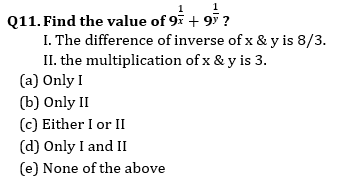Q12. How many women are required to complete the work in 12 days.
I. Efficiency of men are ⅔ rd of efficiency of women.
II. 16 men can complete the same work in 10 days.
(a) Only I
(b) Only II
(c) Either I or II
(d) Only I and II
(e) None of the above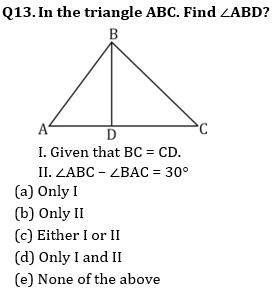Q14. What is the C.I. on a sum at the end of 3 years?
I. C.I. at the end of two years is Rs. 110.
II. Difference between CI and SI at the end of two year is Rs. 100 and rate percent is 10%.
(a) Only I
(b) Only II
(c) Either I or II
(d) Only I and II
(e) None of the above

Q15. Two trains X and Y starts from point A and B respectively towards each other. What is the distance between them when they start?
I. When both trains cross each other, time taken by train X to reach B is twice the time taken by train Y to reach A.
II. Distance between them after 60 min. is 800 km.
(a) Only I
(b) Only II
(c) Either I or II
(d) Only I and II
(e) None of the above

Practice More Questions of Quantitative Aptitude for Competitive Exams:

###### Bank Mains Cracker Study Plan 2021 : Check Now

Solutions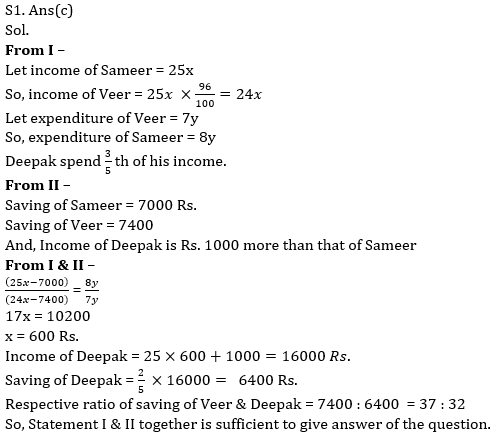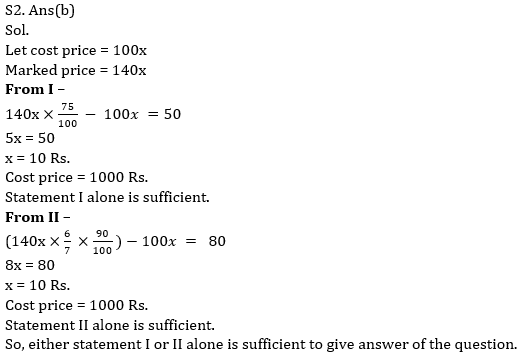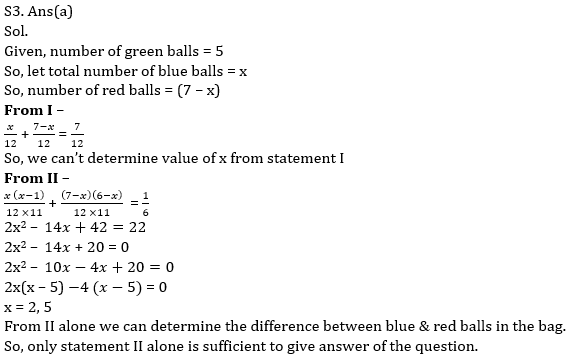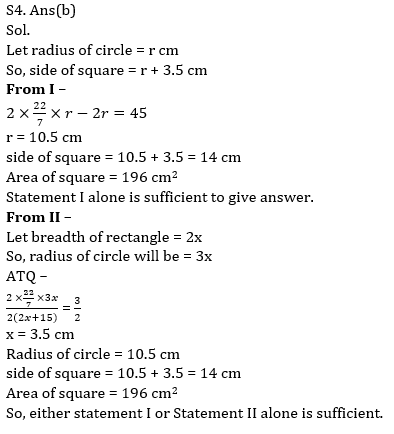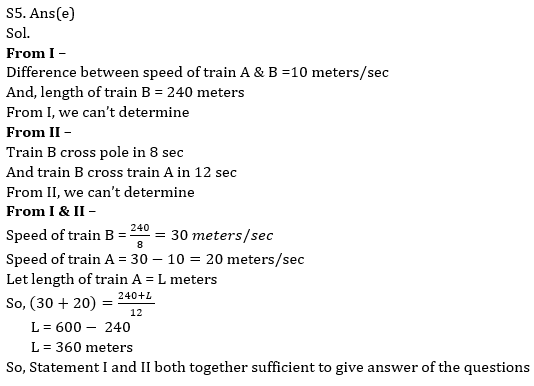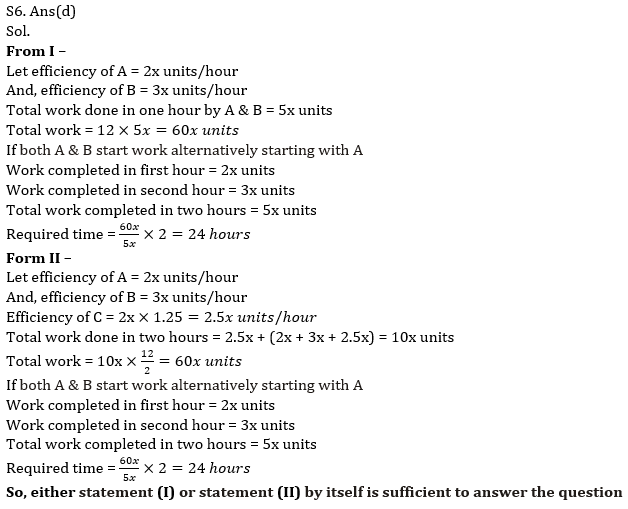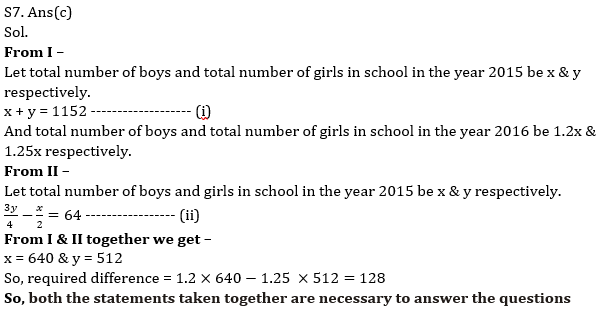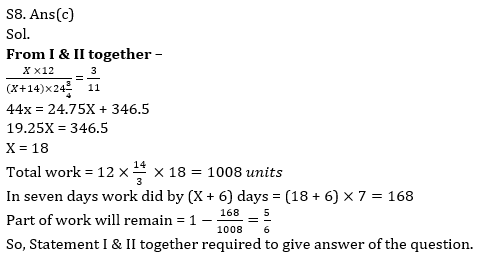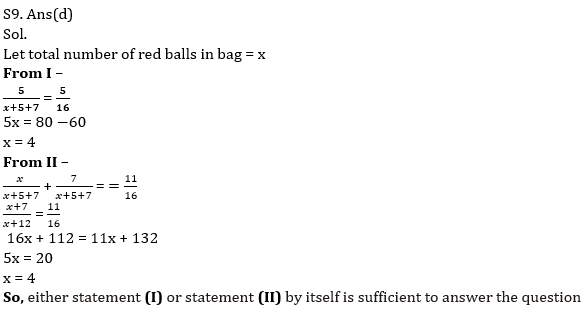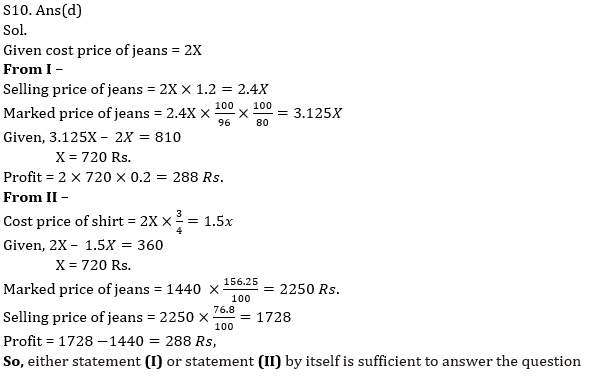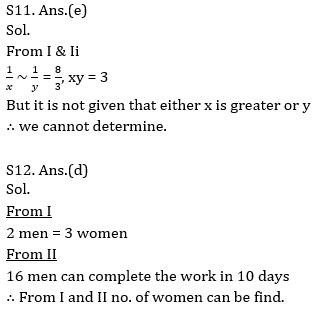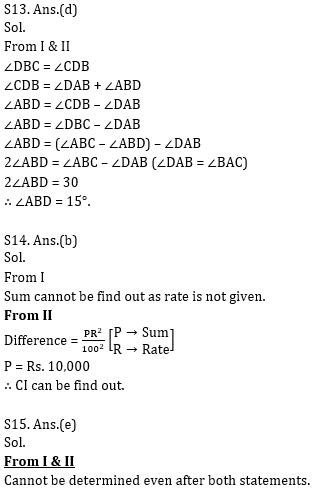Practice with Online Test Series for Bank Mains 2021:×

Thank You, Your details have been submitted we will get back to you.Join India's largest learning destination

What You Will get ?

•Job Alerts
•Daily Quizzes
•Subject-Wise Quizzes
•Current Affairs
•Previous year question papers
•Doubt Solving session

ORJoin India's largest learning destination

What You Will get ?

•Job Alerts
•Daily Quizzes
•Subject-Wise Quizzes
•Current Affairs
•Previous year question papers
•Doubt Solving session

ORJoin India's largest learning destination

What You Will get ?

•Job Alerts
•Daily Quizzes
•Subject-Wise Quizzes
•Current Affairs
•Previous year question papers
•Doubt Solving session

Enter the email address associated with your account, and we'll email you an OTP to verify it's you.Join India's largest learning destination

What You Will get ?

•Job Alerts
•Daily Quizzes
•Subject-Wise Quizzes
•Current Affairs
•Previous year question papers
•Doubt Solving session

Enter OTP

Please enter the OTP sent to
/6

Did not recive OTP?

Resend in 60sJoin India's largest learning destination

What You Will get ?

•Job Alerts
•Daily Quizzes
•Subject-Wise Quizzes
•Current Affairs
•Previous year question papers
•Doubt Solving sessionJoin India's largest learning destination

What You Will get ?

•Job Alerts
•Daily Quizzes
•Subject-Wise Quizzes
•Current Affairs
•Previous year question papers
•Doubt Solving session

Almost there

+91Join India's largest learning destination

What You Will get ?

•Job Alerts
•Daily Quizzes
•Subject-Wise Quizzes
•Current Affairs
•Previous year question papers
•Doubt Solving session

Enter OTP

Please enter the OTP sent to Edit Number

Did not recive OTP?

Resend 60

By skipping this step you will not recieve any free content avalaible on adda247, also you will miss onto notification and job alerts

Are you sure you want to skip this step?

By skipping this step you will not recieve any free content avalaible on adda247, also you will miss onto notification and job alerts

Are you sure you want to skip this step?